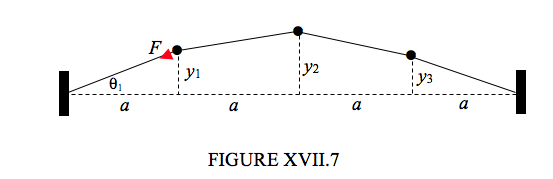$$\require{cancel}$$

# 17.8: Transverse Oscillations of Masses on a Taut String

•• Contributed by Jeremy Tatum
• Emeritus Professor (Physics & Astronomy) at University of Victoria

A light string of length $$4a$$ is held taut, under tension $$F$$ between two fixed points.Three equal masses $$m$$ are attached at equidistant points along the string. They are set into transverse oscillation of small amplitudes, the transverse displacements of the three masses at some time being $$y_1, y_2$$ and $$y_3$$ .

The kinetic energy is easy. It is just

$T = \frac{1}{2} m (\dot{y}^2_1 + \dot{y}^2_2 + \dot{y}^2_3 ). \label{17.8.1}$

The potential energy is slightly more difficult.

In the undisplaced position, the length of each portion of the string is $$a$$.

In the displaced position, the lengths of the four portions of the string are, respectively,

$$\sqrt{y^2+1 + a^2} \qquad \sqrt{(y_2 - y_1)^2+ a^2} \qquad \sqrt{(y_2-y_3)^2+ a^2} \qquad \sqrt{y^2_3 + a^2}$$

For small displacements (i.e. the $$ys$$ much smaller than $$a$$), these are, approximately (by binomial expansion),

$$a + \frac{y^2_1}{2a} \qquad a + \frac{(y_2-y_1)^2}{2a} \qquad a + \frac{(y_2-y_3)^2}{2a} \qquad a + \frac{y^2_1}{2a} \qquad$$

so the extensions are

$$\frac{y^2_1}{2a} \qquad \frac{(y_2-y_1)^2}{2a} \qquad \frac{(y_2-y_3)^2}{2a} \qquad \frac{y^2_3}{2a} \qquad$$

It is also supposed that the tension in the string is $$F$$ and that the displacements are sufficiently small that this is constant. The work done in displacing the masses, which is the elastic energy stored in the string as a result of the displacements, is therefore

$$V = \frac{F}{2a} [y^2_1+(y_2-y_1)^2 + (y_2-y_3)^2 + y^2_3] = \frac{F}{a} (y^2_1+y^2_2 + y^2_3 - y_1y_2 -y_2y_3)$$ .

We note with mild irritation the presence of the cross-terms $$y_1y_2, y_2y_3$$ .

Apply Lagrange’s equation in turn to the three coordinates:

$am\ddot{y}_1 + F(2y_1-y_2) = 0, \label{17.8.3}$

$am\ddot{y}_2 + F(-y_1+ 2y_2- y_3) = 0, \label{17.8.4}$

$am\ddot{y}_3 + F(-y_2+ 2y_3) = 0. \label{17.8.5}$

Seek solutions of the form $$\ddot{y}_1 = - \omega^2y_1, \ddot{y}_2 = - \omega^2y_2, \ddot{y}_2 = -\omega^2 y_3$$.

Then

$(2F- am \omega^2 )y_1 - Fy_2 = 0, \label{17.8.6}$

$-Fy_1 +(2F- am \omega^2 )y_2 - Fy_3 = 0, \label{17.8.7}$

$-Fy_2 +(2F- am \omega^2 )y_3 = 0. \label{17.8.8}$

Putting the determinant of the coefficients to zero gives an equation for the frequencies of the normal modes. The solutions are:

 Slow Medium Fast $$\omega^2_1 = \frac{(2-\sqrt{2}F}{am}$$ $$\omega^2_2 = \frac{2F}{am}$$ $$\omega^2_3 = \frac{(2-\sqrt{2}F}{am}$$

Substitution of these into equations $$\ref{17.8.6}$$ to$$\ref{17.8.8}$$ gives the following displacement ratios for these three modes:

$$y_1: y_2:y_3 = 1: \sqrt{2} :1 \qquad 1:0:-1 \qquad 1: \sqrt{2}:1$$

These are illustrated in Figure XVII.8.

As usual, the general motion is a linear combination of the normal modes, the relative amplitudes and phases of the modes depending upon the initial conditions.

If the motion of the first mass is a combination of the three modes with relative amplitudes in the proportion $$\hat{q}_1:\hat{q}_2: \hat{q}_3$$ and $$\alpha_1:\alpha_2: \alpha_3$$with initial phases its motion is described by

$y_1 = \hat{q}_1 \sin(\omega_1 t + \alpha_1) + \hat{q}_2 \sin(\omega_2 t + \alpha_2) + \hat{q}_3 \sin(\omega_3 t + \alpha_3) \label{17.8.9}$

The motions of the second and third masses are then described by$\sqrt{2} \hat{q}_1\sin(\omega_1 t + \alpha_1) \label{17.8.10}$

and

$y_3 = \hat{q_1}\sin(\omega_1 t + \alpha_1) -\hat{q_2}\sin(\omega_2 t + \alpha_2) + \hat{q_3}\sin(\omega_3 t + \alpha_3). \label{17.8.11}$

These can be written

$y_1 = q_1 + q_2 + q_3, \label{17.8.12}$

$y_1 = \sqrt{2}q_1 - \sqrt{2} q_3 \label{17.8.13}$

and

$y_1 = q_1-q_2 + q_3, \label{17.8.14}$

where the $$q_i$$ , like the $$y_i$$ , are time-dependent coordinates.

We could, if we wish, express the $$q_i$$ in terms of the $$y_i$$, by solving these equations:

$q_1 = \frac{1}{4} (y_1 + \sqrt{2} y_2 + y_3), \label{17.8.15}$

$q_2 = \frac{1}{2} (y_1 - y_3) \label{17.8.16}$

and

$q_3 = \frac{1}{4} (y_1 - \sqrt{2} y_2 + y_3). \label{17.8.17.}$

We have hitherto described the state of the system as a function of time by giving the values of the coordinates $$y_1 , y_2$$ and $$y_3$$. We could equally well, if we wished, describe the state of the system by giving, instead, the values of the coordinates $$q_1 , q_2$$ and $$q_3$$. Indeed it turns out that it is very useful to do so, and these coordinates are called the normal coordinates, and we shall see that they have some special properties. Thus, if you express the kinetic and potential energies in terms of the normal coordinates, you get

$T = \frac{1}{2}m(4\dot{q}^2_1 + 2\dot{q}^2_2 + 4\dot{q}^2_3) \label{17.8.18}$

and

$V = \frac{2F}{a}[(2-\sqrt{2}) q^2_1 +q^2_2 + (2 + \sqrt{2})q^2_3]. \label{17.8.19}$

Note that there are no cross terms. When you apply Lagrange’s equation in turn to the three normal coordinates, you obtain

$am\ddot{q}_1 = -(2 - \sqrt{2})Fq_1, \label{17.8.20}$

$am\ddot{q}_2 = -2Fq_2, \label{17.8.21}$

and

$am\ddot{q}_3 = -(2 + \sqrt{2})Fq_3. \label{17.8.22}$

Notice that the normal coordinates have become completely separated into three independent equations and that each is of the form $$\ddot{q} = - \omega ^2 q$$ and that each of the normal coordinates oscillates with one of the frequencies of the normal modes. Much of the art of solving problems involving vibrating systems concerns identifying the normal coordinates.